#### 期刊菜单

Research on the Mechanism and Control Method of Hollow Conical Light in Biaxial Crystal
DOI: 10.12677/APP.2020.103022, PDF , HTML, XML, 下载: 548  浏览: 830

Abstract: The phenomenon of cone refraction was proposed by Hamilton in 1832 from the perspective of wave theory of light. He predicted that when the light wave propagates along an optical axis of a biaxial crystal, the transmitted light energy will be distributed on a conical surface. This was later confirmed experimentally by Lloyd. According to the cone refraction theory, if a laser beam propagates along one optical axis of biaxial crystal, it will produce hollow cone light. Different from the solid laser, the whole energy of the hollow conical light is distributed on the funnel-shaped conical surface. The energy distribution characteristics of the hollow conical light make the hollow conical light have advantages and application prospects that the solid laser can’t match. For example, the retractable characteristics of the hollow conical light make it different from the solid laser in principle in scanning point by point and line by line, with scanning. With the advantages of fast tracing speed and wide scanning range, hollow conical light can be used for multi-target detection and tracking. Therefore, hollow conical light has a wide range of applications in printing, scanning, copying, radar, guidance, communication, disease diagnosis and other industrial, medical and national defense fields.

1. 引言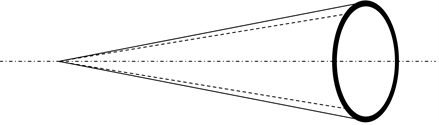Figure 1. Schematic diagram of hollow conical light

1816年，菲涅耳提出了他的行波理论，这个理论是在惠更斯的波前分布实验的基础上提出的，短短几年之后，他通过实验发现了波动理论中的衍射和折射现象。

1832年，Hamilton用几何光学方程把菲涅耳的波动理论和牛顿的微粒理论结合了起来他把光线描述成具有某些特性的函数等位面的法线，并预测了锥形折射现象，这个现象，是菲涅耳的波理论四阶近似的结果Hamilton指出，在一个双轴介质中，折射率表面有两层，对应由双折射产生的两束光线，旋转椭球的两层表面与两条光轴相交于四个点。

1833年，Lloyd证实了Hamilton对锥形折射的预测，他在实验中发现空心光环的偏振是线性的，沿光轴旋转一周，偏振方向只旋转半周，他还提出在无旋光的透明双轴晶体中，锥形折射模式可以由一个无量纲参数描述，即出射光环的半径和入射光宽度的比值。

1841年，Potter用霞石进行了内锥折射的实验，实验中Potter发现了在晶体的焦像平面的重要性，因为这里出现光源的会聚像，他发现在这个平面外的光锥截面处是由两个同心环构成的。

1549年，Poggendorf根据Potter的实验，提出锥形折射的亮环实际上是一对同心亮环，它们之间有一个暗环，1855年，Haidinger进行了实验，证实了这一点。

1567年，Stokes通过对Bessel方程的研究，得到了现在用来理解锥形衍射的渐近理论，并由此提出了渐近的积分形式，指出只有通过旁轴近似才能充分描述锥形折射的衍射现象。

1941年，Raman首先提出了内锥折射和外锥折射的联系，他描述了远场处锥形折射光环的模式，描述了随着与焦平面距离的增加，锥形折射光环的变化，由于两片波表面分离，每个面上的环分离并且扩散。

1978年，Belskii和Khapalyuk提出了双轴晶体内圆偏振光的衍射积分，后来他们又进一步发展了这个理论，并提出旁轴衍射理论可以用于旋光晶体，得到了由一个小孔产生的光束入射薄晶体形成的锥形衍射积分，并获得了其Legendre展式1997年，WarniCk和Arnold通过数学计算，运用Belskii和Khapalyuk的理论，由动态格林函数表示电场，解释了Schell和Bloembergen的实验现象，他们还模拟了晶体外亮环的能量分布，从中发现内环的次级环结构。

1997~1999年，长春理工大学宁国斌、梁柱教授等对激光的动态外锥折射进行了一系列的实验研究，并且取得了丰富的研究成果，他们提出了双轴晶体锥形折射的控制技术，并获得了锥角可控的动态外锥折射，研制出了一种新型的激光扫描器。

2002年，李华、李强等通过晶体内光波的简正模方程，得到了当光线沿晶体内的光线光轴传播时，在双轴晶体中的电场强度和电位移矢量的表达式。

2003年，薛冬、李国华等通过几何方法和理论推导，得到了双轴晶体中的波法线光轴与光线轴方向，并印证了它们是两个完全不同的光轴，其空间位置有差别。

2005年，孟繁华、宋连科等利用光波面和光波法线面的曲线方程，得出了在光波面上，光轴角度和光线轴角度的表达式，并根据锥形折射的性质及对应光波面上的位置关系，推导了内锥折射锥顶角和外锥折射锥顶角的表达式。

2. 双轴晶体在应用中的技术需求

1. 在光扫描方面。由于空心圆锥形光是控制锥角的大小从而进行扫描，锥角缩放一次就能对目标扫描两次，形成两帧图像，因此，相对于实心光束，空心圆锥形光的扫描范围更广，速度更快，也不会产生遗漏，为扫描领域提供了一种快捷、高效的光学扫描方式  。如果在空心圆锥形光扫描范围之内有多个目标，则利用CCD可以同时对多个目标进行成像。因此，空心圆锥形光扫描可以用于印刷、复印、医疗、光学检测和激光布景等方面，也可以用于形成大气云图与地球表面的图像  。

2. 在光探测方面。与实心光束相比，空心圆锥形光和有着两个方面的优势：一是由于空心圆锥形光的能量分布在整个漏斗形圆锥面上，因此，光束探测面远大于实心光束，只要空心圆锥形光具有较大的探测空间；二是通过对空心圆锥形光锥角的控制，可使扫描范围进一步扩大，进而实现对目标的搜索和跟踪。因此，空心圆锥形光克服了现有激光雷达的不足，可以同时完成对多个目标的速度和距离的测量，并可以对多个目标进行跟踪和成像。

3. 在制导方面。

3. 实验方案

${\chi }_{0}=\frac{{n}_{y}^{2}}{2}\left(\frac{1}{{n}_{x}^{2}}-\frac{1}{{n}_{z}^{2}}\right)\mathrm{sin}2\beta =\frac{\sqrt{\left({n}_{y}^{2}-{n}_{z}^{2}\right)\left({n}_{z}^{2}-{n}_{y}^{2}\right)}}{{n}_{x}{n}_{z}}$ ，我们能够得到KNbO3产生的内锥折射角度为3.83˚，可以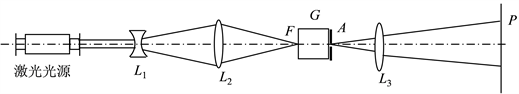Figure 2. Schematic diagram of the generation and control system of the cone light with the center of time and space refracted by the external cone of biaxial crystal

3.1. 内锥形折射方案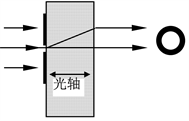Figure 3. Inner cone refraction diagram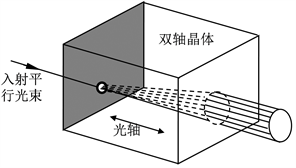Figure 4. Inner cone refraction

3.2. 外锥形折射方案Figure 5. Schematic diagram of external cone refraction

3.3. 双轴晶体内锥折射时锥角控制方案

3.4. 实验现象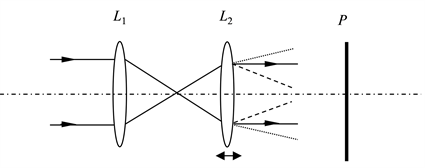Figure 6. Control diagram of light cone angle of hollow cone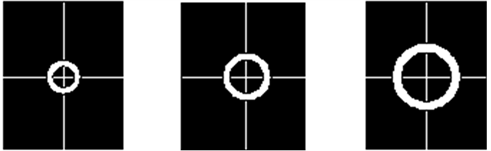Figure 7. Change of aperture size of ring at different times in the same position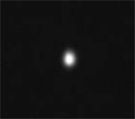(a)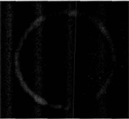(b)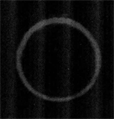(c)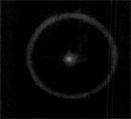(d)

Figure 8. Experimental effect

4. 双轴晶体产生锥形光的机理研究

$\frac{{x}^{2}}{{n}_{x}^{2}}+\frac{{z}^{2}}{{n}_{z}^{2}}=1$ (1)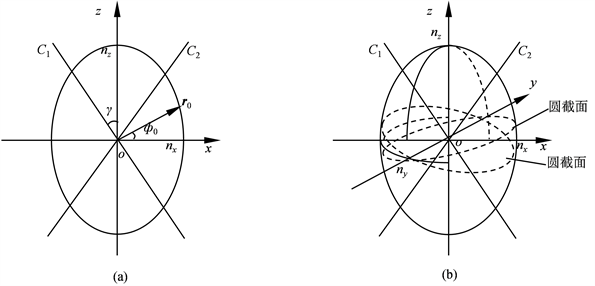Figure 9. xz section and optical axis of index ellipsoid of biaxial crystal

$\frac{{\left({n}_{y}\mathrm{cos}{\varphi }_{0}\right)}^{2}}{{n}_{x}^{2}}+\frac{{\left({n}_{y}\mathrm{sin}{\varphi }_{0}\right)}^{2}}{{n}_{z}^{2}}=1$ (2)

$\text{tg}{\varphi }_{0}=\text{tg}\gamma =±\frac{{n}_{z}}{{n}_{x}}\sqrt{\frac{{n}_{y}^{2}-{n}_{x}^{2}}{{n}_{z}^{2}-{n}_{y}^{2}}}$ (3)

4.1. 探讨锥形折射的能量传输过程，及各参数对其能量分布的影响

$I=\alpha \left({\theta }_{1}\right)2\pi {\theta }_{1}\Delta {\theta }_{1}$ (4)

${I}_{1}={\alpha }_{1}\left({\theta }_{1}\right)2\pi \left({\gamma }_{0}±{\theta }_{1}\right)\Delta {\theta }_{1}$ (5)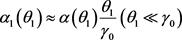(6)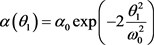(7)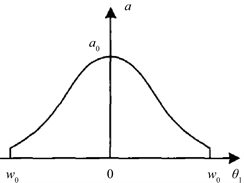(a)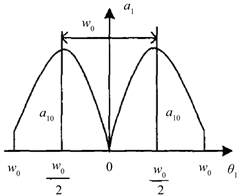(b)

Figure 10. Energy distribution of non parallel incident Gaussian beams along the ring

4.2. 通过推导得到的结论，研究锥行折射产生的光环的精细结构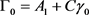(8)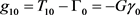(9)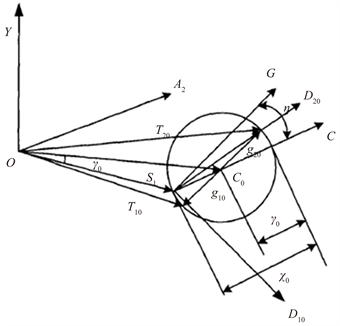Figure 11. Schematic diagram of conic refraction vector relationship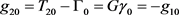(10)

5. 总结

  Wang, X. and Littman, M.G. (1993) Laser Cavity for Generation of Variable-Radius Rings of Light. Optics Letters, 18, 767-768. https://doi.org/10.1364/OL.18.000767  Rioux, M., Belanger, P.A. and Cormier, M. (1977) High-Order Circular-Mode Selection in a Conical Resonator. Applied Optics, 16, 1791-1792. https://doi.org/10.1364/AO.16.001791  Tamm, C. and Weiss, C.O.J. (1990) Bistability and Optical Switching of Spatial Patterns in a Laser. Journal of the Optical Society of America B, 7, 1034-1037. https://doi.org/10.1364/JOSAB.7.001034  Allen, L., Beijersbergen, M.W., Spreeuw, R.J.C., et al. (1992) Orbital Angular Momentum of Light and the Transformation of Laguerre-Gaussian Laser Modes. Physical Review A, 45, 8185-8189. https://doi.org/10.1103/PhysRevA.45.8185